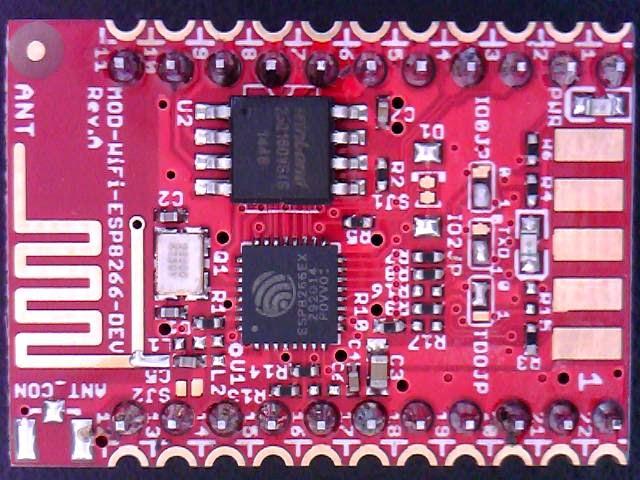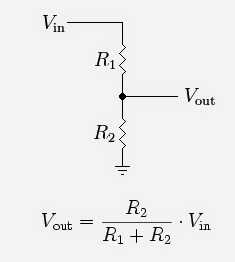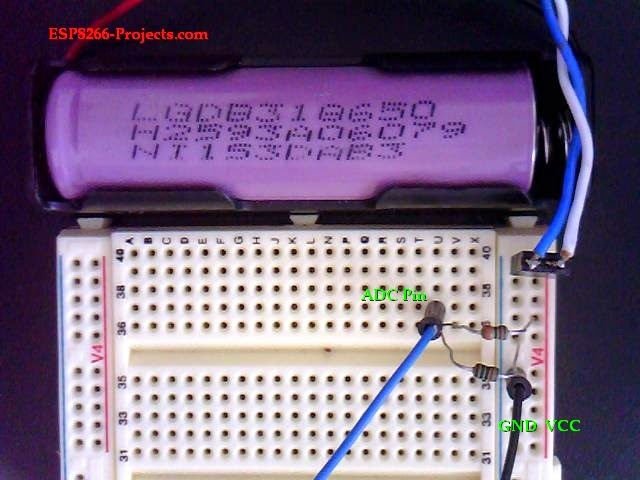## Wednesday, March 18, 2015

----------------------------------  UPDATE  --------------------------------------

For a ADC Input Frontend with Auto-range capabilities in the 0-40V Input range take a look also at the new ADC Input related article

Added also a new example for a 0-5V input range with Voltage divider and LSB calculation, "the easy way": ESP8266 internal ADC 2 - the easy way example

---------------------------------------------------------------------------------------

After talking in the previous article about DAC (Digital to analog conversion) let's take a look also to the ADC (Analog to Digital Conversion) process story.

What is the purpose of an ADC ?
The ADC translates an analog input signal to a digital output value representing the size of the input relative to a reference.

One of the good news about is that ESP8266 has an ADC inside and at least ESP-07, ESP-12, Olimex MOD-WIFI-ESP8266-DEV modules have the ADC Pin available.ESP8266 - ESP-07 ModuleOlimex MOD-WIFI-ESP8266-DEV

Some not so good news about ESP8266 internal ADC, at least until now:
•   Documentation available it's at a very poor level. More like a leaflet combined with a marketing presentation. If you were able to find anything more than this one please feel free to share.
•  Only one ADC, one analog input pin, resolution probably determined by the ESP8266 community as been a 10 Bit one thru trial and error or some internal data leakage and has a not so clear range from 0 to about 1V. No other technical specifications. None. Engineering fun :)

So, the legitimate Question arrived: it is any good for anything or just a fancy added function for marketing purposes ? Well...will see below

The first simple application that has come in my mind was to use the new builded Li-ion Battery Module to power up ESP8266 module and in the same time to read the Li-ion Cell voltage thru a long period of time. A voltage logger for the Li-ion Cell if you want. 10 Bits resolution must be enough for the purpose of the experiment, to see some trending data for the Li-ion Cell.

What we will need:
• CBDB Board
• USB adapter (take a look on Part 1 for details how to connect them together)
• Voltage Divider as below

General considerations :

As maximum voltage input is expected to be 1V only and because our Li-ion Cell fully charged voltage goes up to 4.2-4.3V it's obviously that we need to find a way to "translate" the voltage domain between 0-4.3V to 0-1V. They are many different techniques available for doing that but the easiest one and the one that we will use here is the Resistive Voltage Divider (RVD).

A RVD (also known as a potential divider) is a passive linear circuit that produces an output voltage (Vout) that is a fraction of its input voltage (Vin). Voltage division is the result of distributing the input voltage among the components of the divider.

We will use for our project the simpler example of a voltage divider: two resistors connected in series, with the input voltage applied across the resistor pair and the output voltage emerging from the connection between them.Voltage divider schematic and Vout formula

The result:Voltage divider

• use high quality resistors, 1% or less with as small as possible ppm/C. In this case were used metal film resistors, 1%, 50ppm/C. Try to avoid carbon ones.
• avoid long wires that can produce itself a voltage drop due to internal resistance. For a quick explanation and calculation take a look here and for PCB traces here
• yes, I know, pedants might say breadboard is not a good idea for something like that but believe me, in this case, for a quick test, will make no difference. It's a 10 Bit ADC and we are doing trending measurement.

Let's find out what resistor values we will need for our RVD:
• Vinmax = 4.3 V
• Voutmax = VADCin_max = 1V
•  Vout=Vin*R2/(R1+R2)
From the resistor ratio calculation choosed values are: R1=33K and R2=10k. You can choose also other values as long as you keep accurate the ratio between, better go upper that that. 330k and 100k should give the same result for example.

Software implementation

For programming CBDB Board and uploading the driver and the software we will continue to use the LuaUploader as before.

We will implement 3 different type of ADC Read functions,  to see if any notable difference:

end

i=0
while (i<10) do
i=i+1
end
end

As probably Read_ADC() and Read_AVG() are pretty straigh forward things I will not insist on them. Read_DCM() function it's a little bit special, as is using a special technique called oversampling and decimation.

The theory behind oversampling and decimation is rather complex, but using the method is fairly easy. The technique requires a higher amount of samples. These extra samples can be achieved by oversampling the signal. For each additional bit of resolution, n, the signal must be oversampled four times. To get the best possible representation of a analog input signal, it is necessary to oversample the signal this much, because a larger amount of samples will give a better representation of the input signal, when averaged.

That means that in our case if we want to increase resolution from 10 to 12 bit we will need to take 16 samples.

Another requirement to make this method work properly is that the signal-component of interest should not vary during a conversion. However another criteria for a successful enhancement of the resolution is that the input signal has to vary when sampled. This may look like a contradiction, but in this case variation means just a few LSB. The variation should be seen as the noise-component of the signal. When oversampling a signal, there should be noise present to satisfy this demand of small variations in the signal.

i=0
while (i<16) do
i=i+1
end
end

Some first time run results:

srv=net.createServer(net.TCP)
srv:listen(80,
function(conn)
conn:send("HTTP/1.1 200 OK\n\n")
conn:send("<META HTTP-EQUIV=\"REFRESH\" CONTENT=\"5\">")
conn:send("<html><title>LOG Server - ESP8266</title><body>")
conn:send("<h1>Data Logger Server - ESP8266</h1><BR>")

" V</font></b><br>")

" V</font></b><br>")

" V</font></b><br>")
conn:send("<BR><BR><BR>Node.HEAP     : <b>" .. node.heap() .. "</b><BR>")
conn:send("IP ADDR    :<b>".. wifi.sta.getip() .. "</b><BR>")
conn:send("TMR.NOW    :<b>" .. tmr.now() .. "</b><BR<BR><BR>")
conn:send("</html></body>")
conn:on("sent",function(conn) conn:close() end)
conn = nil
end)
end)

Test Video:

ilja.bobkevic said...

Hi,

Thanks you very much for this post! It's really a good reference getting started. However I have a few questions regarding calibration value calculations. Could you please elaborate a bit mor on 4/978 and 0.001020880. Where does it come from?

Thank you!

Unknown said...

In case of the READ_ADC() function, calibration is based on voltage divider AND Vref, voltage divider value = 4 and Max ADC input Voltage 0.978 (based on the available Vcc 3.276 and corresponding Vref value)

In case of the readADC_dcm() function, using the oversampling and decimation technique with a target resolution of 12bit, 0.001020880 represents the calculated LSB in this case.

Unknown said...

Great post! - ESP-12 at least has a voltage divider to map 3.3 to internal 1 V - This is something that was very undesireble in my case, but I found a workaround.

I was trying to interface a lm35dz which does 10mV/ºC and I didnt want the loss of resolution. I found out the voltage divider has it's mid point (the one that goes into the adc) exposed in the "RSV" - reservd pin imediatly next to A0 pin, so anyone can chose to use the 1.0V upper limit or 3.3V by chosing which pin to connect. You'll still have a 100k resistor from this RSV pin to ground. If your impedance is not very large you can ignore this, I chose to remove the resistor, giving you a direct access to the actual module adc pin.

Unknown said...

my adc seems to jut give me

These even if i have nothing going to ADC or more than a volt to ADC..

nhuythuy said...
This comment has been removed by the author.
nhuythuy said...

I tested and found that the max value that I can have from analog input pin (A0) is 1024 (not 1023) when I connected to 3.3V on the NodeMCU board:

Rocco said...

To measure up to 15V what resistors should i use?

Unknown said...

Rocco, for any desired Input Voltage range, just read above and apply the formula: Vout=Vin*R2/(R1+R2).

For example, in case of Vin=15V, let's say we choose R1=1Meg, then for R2 we will obtain 67k -> Vout = 0.942, exactly what we are looking for.
Same Vout value will be also when R1=100k and R2=6k7. But different input impedance. Choose values also based on that. Also as a good practice, a input buffer in front of the ADC will be a good idea.

Unknown said...

I'm also curious about the 4/978 values - I understand 978 is for the scaled max ADC input voltage, but where exactly does the 4 come from?

Unknown said...

Max ADC input Voltage = 0.978 - based on the measured Vcc 3.276 and corresponding Vref value.

4/978 = calculated and calibrated VDivider output LSB based on the real values of the Vin, Vout and resitor divider values.

Calculation example

Teoretical :
Max Input Voltage = 4.21V

Rdiv = 33k/10k -> 4.21V input -> 0.979V Output = Max ADC Input Voltage

Divider ratio = 4.300306435
Voltage Divider LSB = 0.004111328

To make it even easier:

Direct Measured Values:

Max Input Voltage = 4.188139 ->
-> Voltage Divider LSB = 0.00409
4/978 = 0.00408998 == Voltage divider LSB.

Unknown said...

My example:

Max Input Voltage = 14.5V

Rdiv=47k/3.4k -> 14.5V input-> 0.978V Output = Max ADC Input Voltage

Divider Ratio = 14.5V/.978V = 14.8262
Voltage Divider LSB = Divider ratio * ADC LSB

Also can you please define LSB?

Unknown said...

Hi David,

Least Significant Bit (LSB)

Definition:

In a binary number, the LSB is the least weighted bit in the group. Typically, the LSB is the furthest right bit. For an ADC or DAC, the weight of an LSB equals the full-scale voltage range of the converter divided by 2N, where N is the converter's resolution.

OR, if you want in simple terms, is the smallest possible Voltage that can be represented for the given ADC resolution.

In our case, for a 10Bit ADC:
Full scale voltage (FsV) = 0.978V
10Bit -> 2^10 = 1024
LSB = FsV/1024 = 0.000956055V

For you requested example:

Max Input Voltage = 14.5V
Rdiv = 47k/3.4k

Doing the math, indeed, the resitior divider you choose will give you the correct ADC input maximum voltage of 0.978V.

Now, to calculate the LSB (or the smallest step value if you want) for your new full input range of 14.5V:

Your divider Ratio is : 14.82618

Then Max Input Voltage LSB (let's name it simple Vi_LSB):

Vi_LSB = ADC LSB * Divider ratio
Vi_LSB = 0.000956055 * 14.82618 =
Vi_LSB = 0.014174635V = 14.174635mV

=> the smallest Input Voltage step that you can read is about 14.175mV.

This will be also your resolution (in Volts) for a Voltage range of 0-14.5V using the given Resitor Divider from above and the ESP8266 10bit ADC with a max voltage input of 0.978V.

Unknown said...

Hey Tracker J,

Thanks for the help on the voltage measurement, that's reading fairly consistently now!

Unfortunately, I'm having another issue with the ESP8266 Huzzah that I'm wondering if you have encountered before. The ESP is either resetting itself or running the code improperly - I'm not sure. I'm using the Arudino IDE for the ESP and this is my first time working with ESPs in general. To counter the resets I tried using yields and delays in the code along with large capacitors in the circuit to minimize voltage fluctuations. These have not proved successful.

If I send you the source code, could you possible take a quick look at it? It seems like you have lots of experience with ESPs and could give me some valuable information. This would really go a long way!

Unknown said...

Hi David,

I don't have a Huzzah but you can send me the code to take a look at tech (at) esp8266-projects.com.

KevinR said...

I may be dumb, but is there a reason why your calculation of divider ratios is always exactly 1 more than I am calculating?

Teoretical :
Max Input Voltage = 4.21V

Rdiv = 33k/10k -> 4.21V input -> 0.979V Output = Max ADC Input Voltage

Divider ratio = 4.300306435 (But 33k/10k = 3.3)

The other examples in the comments are yielding the same difference of 1.

Unknown said...

Hi Kevin,

If you read carefully above you will see that the formula for calculate the Vout from a Resistive Divider, based on R1,R2 and Vin is

Vout = (R2/(R1+R2))*Vin

So,

For R1 = 33k, R2=10k and Vin=4.2V
-> Vout = 0.978
Vin/Vout = 4.2/0.978 = 4.3
Vout is 4.3 times smaller that the Vinput and this is the divier ratio.

Unknown said...

David, I had the same problem with the esp8266, adding a 470 uF capacitor before the esp made my problems go away

Unknown said...

Hi Tracker,

Thank you for the good review. I am not sure I quite understand all the terminology or whether I am doing something wrong with my test.

I have a Vin max of 5v

My RVD = 5 x (16k/(68k+16k))=0.952

So my voltage divider ratio is 5/0.952=5.25

When I measure the actual voltage to the ADC pin I get 0.680??? why is there such a big difference between RVD voltage and my actual voltage?

In any case, my sketch reads 633 on A0. So if I understand correctly from the readADC() function, this translates to 633x5.25/680 = 4.89v

Is this correct? I find 110mV a big difference.

Mike

Unknown said...

the voltage divider calculation looks correct: 0.952 = MAX ADC input voltage for a Vin = 5Vcc MAX
What represents 0.680? Volts for a 5V input voltage thru Volgate divider? This is a read value with a multimeter or thru ESP ADC and some software calculations?
And 633?

Can you please post the code that you are using somewhere to take a look. How are you calculating the LSB?
In your above case it should be:
LSB = MaxInputVoltage/1024
Be aware ! MaxInputVoltage is NOT RVD!!
MaxInputVoltage = the maximum voltage that ADC can read and return a valid value! You need this value for proper calibration.

Unknown said...

Hi Tracker,

The 0.680v value is actualy measured with a meter at the voltage divider when 5v is applied.

I think my problem is this:

MaxInputVoltage = the maximum voltage that ADC can read and return a valid value! You need this value for proper calibration.

How do I find the MaxInputVoltage? is this alway 0.978v (what you call Full scale voltage)?

My sketch is very simple for now.

adcV = av * 5.25/680; I'm sure 680 is wrong
serialprint("A0: ");
serialprintln(av);
serialprint("Voltage: ");
}

I call this every 10 seconds in the main loop

633 is the actual value I get from analogRead(A0)

Thank you,

Mike

Unknown said...

In my function 4/978 = calculated and calibrated VDivider output LSB based on the real values of the Vin, Vout and resitor divider values.

Let's try a bit simpler I think:

MaxInputADCVoltage (named also Full Scale Voltage) = the voltage that you measure with your Voltmeter at the ADC input pin when reading the maximum possible ADC value that is 1023. If you go higher with your Input Voltage you will be out of domain > 1024!

In my particular case, for a VCC = 3.276V the MaxADCInputVoltage (or FSV) = 0.978V

After you have your own MaxInputValue you can calculate your LSB and from there just apply the formula

You can check it anytime using a known (measured with Voltmeter) Voltage input value.

Please look above at the example I've done for David, for his 14.5V Voltage input.

Unknown said...

Tracker,

Yes ... a bit simpler is good :)

I had looked at that example and this is where I get confused.

Just now you say that the MaxInputADCVoltage (named also Full Scale Voltage) = the voltage that you measure with your Voltmeter at the ADC input pin when reading the maximum possible ADC value that is 1023. If you go higher with your Input Voltage you will be out of domain > 1024!

However, when I look at David's example I get the impression that the MaxInputADCVoltage is a calculated value as stated in this comment:

"Doing the math, indeed, the resitior divider you choose will give you the correct ADC input maximum voltage of 0.978V."

In any case, I think what you are saying is I have to increase Vin until my ADC reading is at 1023, once I get there I measure, with a voltmeter, the MaxInputADCVoltage at the ADC pin. My Vin will likely be higher than my intended 5v max Vin, does that matter? Is this what I have to do?

Sorry for all the question, normally I catch on quite fast.
Mike

Unknown said...

Tracker,

One more thing. Why is it that my meter reading at the ADC pin is 0.680v when I apply 5v to the RVD. I would have though is would be closer to what I calculated.

RVD = 5 x (16k/(68k+16k))=0.952

Mike

Unknown said...

Hi Mike,

To make it even simpler, I will do right now a new post with a new example, just give me 10 mins. It's quite hard to follow here in the comments.

See you on the new post page to follow.

Unknown said...